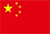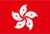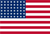Scanning QR Code• Online Inquiry

+
Congratulations
You've successfully got advanced quotes worth 5000 HKD
• Home
• Quotes
• News
• Community
• Learn
• Help Center
• Language
•••EN

# Position field introduction

### 1. Cost price (diluted cost)

Formula: Cost price = (total amount bought during the holding period-total amount sold during the holding period) /number of holdings

Meaning: Refers to the guaranteed price (excluding commissions and fees) of a stock during the holding period at which the user can break even by selling at that value. The profit and loss of each transaction during the holding period (excluding cash dividends, rights issues, etc.) are calculated, taking into account both purchases and changes in sales.

### 2. Amount of profit and loss

Formula: Amount of profit and loss = (current price-cost price) × number of holdings

Meaning: refers to the unclosed profit and loss during the holding period of a stock.

### 3. Profit and loss ratio

Formula: P/L ratio = P/L amount / (cost price x number of holdings) = (current price - cost price) / cost price

Meaning: the ratio of the profit/loss amount to the total cost.

When the cost price is negative, the calculation is no longer applicable, so the P/L ratio is shown as 0.00%. This is also a common practice among peer brokerage firms that use diluted costs as their cost price.

### 4. Floating profit and loss today

Formula: today's floating profit and loss = (today's market value - yesterday's market value) + (today's selling turnover - today's buying turnover)

Meaning: refers to the user's profit and loss from the previous trading day to the settlement of the current trading day.

### 5. Open position ratio

Formula: Open position ratio = market value of single position / net account value

Meaning: The ratio of a user's position to the NAV of an account.

Note: In the client terminal, users can understand account information from different perspectives by switching the denominator (single/overall account equity) of the position accounted for.

### 6. Holding period

Meaning: The complete and continuous period of time from the opening of a stock position to its liquidation.

If a stock is liquidated at some point and then bought or transferred into a new position, it is considered a new position and a new holding period begins. The cost of each holding period and the profit or loss is calculated independently.

## Position display settings

You can use the following methods to set the position page field layout.

Method one: In the [My] page, click [Settings]> [Transaction Settings]> [Position Display] to enter the corresponding page.

Method Two: In the [Trading] page, click [Position sorting symbol]> [Position display] to enter the corresponding page.

Back to the Top